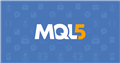# How can i draw arrow & alert in an indicator after candle close

any expert please help me my indicator show me arrow & alert when candle start but i want that arrow & alert show after candle close. if any expert help me i will share code & indicator screen shot also.

```double hma[];
double arrowUp[];
double arrowDn[];
double work[];
double trend[];
double         ExtBuffer0[];
double         ExtBuffer1[];
double         ExtBuffer2[];
double         ExtBuffer3[];
double         ExtBuffer4[];
double         ExtBuffer5[];
double         ExtBufferh1[];
double         ExtBufferh2[];

int    HalfPeriod;
int    HullPeriod;

string indicatorFileName;
bool   returnBars;
bool   calculateValue;
int    timeFrame;
//--------------------------------------------------------
int init()
{
IndicatorBuffers(15);
SetIndexBuffer(0,hma);
SetIndexStyle(0,DRAW_ARROW);
SetIndexArrow(0,159);
SetIndexStyle(1,DRAW_ARROW);
SetIndexArrow(1,159);
SetIndexBuffer(3,arrowUp);
SetIndexBuffer(4,arrowDn);
SetIndexBuffer(5,trend);
SetIndexBuffer(6,work);
SetIndexBuffer(7,ExtBufferh1);
SetIndexBuffer(8,ExtBufferh2);
SetIndexBuffer(9,ExtBuffer3);
SetIndexBuffer(10,ExtBuffer4);
SetIndexBuffer(11,ExtBuffer5);
SetIndexBuffer(12,ExtBuffer0);
SetIndexBuffer(13,ExtBuffer1);
SetIndexBuffer(14,ExtBuffer2);

if (ShowArrows)
{
SetIndexStyle(3,DRAW_ARROW,0,5); SetIndexArrow(3,241);
SetIndexStyle(4,DRAW_ARROW,0,5); SetIndexArrow(4,242);
}
else
{
SetIndexStyle(3,DRAW_NONE);
SetIndexStyle(4,DRAW_NONE);
}
//---------------------------------------------------------------
HMAPeriod  = MathMax(2,HMAPeriod);
HalfPeriod = MathFloor(HMAPeriod/HMASpeed);
HullPeriod = MathFloor(MathSqrt(HMAPeriod));

indicatorFileName = WindowExpertName();
calculateValue    = TimeFrame=="calculateValue"; if (calculateValue) { return(0); }
returnBars        = TimeFrame=="returnBars";     if (returnBars)     { return(0); }
timeFrame         = stringToTimeFrame(TimeFrame);
//--------------------------------------------------------------------------------------------
IndicatorShortName("CI_01 ");
return(0);
}

void deinit()
{
deleteLines();
}
void deleteLines()
{
int lookForLength = StringLen(UniqueID);
for (int i= ObjectsTotal()-1; i>=0; i--)
{
string name = ObjectName(i);
if (StringSubstr(name,0,lookForLength)==UniqueID) ObjectDelete(name);
}
}
int start()
{
int    limit;
double Value=0,Value1=0,Value2=0,Fish=0,Fish1=0,Fish2=0;
double price;
double MinL=0;
double MaxH=0;
int i,counted_bars = IndicatorCounted();
if(counted_bars<0) return(-1);
if(counted_bars>0) counted_bars--;
limit=MathMin(Bars-counted_bars,Bars-1);
if (returnBars) { hma = MathMin(limit+1,Bars-1); return(0); }
for(i=0; i<limit; i++)
{  MaxH = High[Highest(NULL,0,MODE_HIGH,period,i)];
MinL = Low[Lowest(NULL,0,MODE_LOW,period,i)];
price = (High[i]+Low[i])/2;
Value = 0.33*2*((price-MinL)/(MaxH-MinL)-0.5) + 0.67*Value1;
Value=MathMin(MathMax(Value,-0.999),0.999);
ExtBuffer0[i]=0.5*MathLog((1+Value)/(1-Value))+0.5*Fish1;
Value1=Value;
Fish1=ExtBuffer0[i];
if (ExtBuffer0[i]>0) ExtBuffer1[i]=10; else ExtBuffer1[i]=-10;
}
for(i=limit; i>=0; i--)
{
double sum  = 0;
double sumw = 0;

for(int k=0; k<smooth && (i+k)<Bars; k++)
{
double weight = smooth-k;
sumw  += weight;
sum   += weight*ExtBuffer1[i+k];
}
if (sumw!=0)
ExtBuffer2[i] = sum/sumw;
else  ExtBuffer2[i] = 0;
}
for(i=0; i<=limit; i++)
{
sum  = 0;
sumw = 0;

for(k=0; k<smooth && (i-k)>=0; k++)
{
weight = smooth-k;
sumw  += weight;
sum   += weight*ExtBuffer2[i-k];
}
if (sumw!=0)
ExtBuffer3[i] = sum/sumw;
else  ExtBuffer3[i] = 0;
}
for(i=limit; i>=0; i--)
{
ExtBuffer4[i]=EMPTY_VALUE;
ExtBuffer5[i]=EMPTY_VALUE;
ExtBufferh1[i]=EMPTY_VALUE;
ExtBufferh2[i]=EMPTY_VALUE;
if (ExtBuffer3[i]>0) { ExtBuffer4[i]=ExtBuffer3[i]; ExtBufferh1[i]=ExtBuffer3[i]; }
if (ExtBuffer3[i]<0) { ExtBuffer5[i]=ExtBuffer3[i]; ExtBufferh2[i]=ExtBuffer3[i]; }
}
{
for(i=limit; i>=0; i--) work[i] = 2.0*iMA(NULL,0,HalfPeriod,0,MODE_LWMA,HMAPrice,i)-iMA(NULL,0,HMAPeriod,0,MODE_LWMA,HMAPrice,i);
for(i=limit; i>=0; i--)
{
hma[i]   = iMAOnArray(work,0,HullPeriod,0,MODE_LWMA,i);
trend[i] = trend[i+1];
if (hma[i] > hma[i+1]) trend[i] =  1;
if (hma[i] < hma[i+1]) trend[i] = -1;
if (trend[i]!= trend[i+1])
if (ExtBuffer3[i]>1)
arrowUp[i] = hma[i] - iATR(NULL,0,+80,i)/1.0;
else
if (trend[i]!= trend[i-1])
if (ExtBuffer3[i]<1)
}
{
if (trend[i]!= trend[i+1] && (ExtBuffer3[i]>0))
arrowUp[i] = High[i+1];

if (trend[i]!= trend[i-1] && (ExtBuffer3[i]<0))
arrowDn[i] = Low[i+1];
}

return(0);
}
int shift = -1; if (ArrowsOnCloseBar) shift=1;
limit = MathMax(limit,MathMin(Bars-1,iCustom(NULL,timeFrame,indicatorFileName,"returnBars",0,0)*timeFrame/Period()));
for (i=limit; i>=0; i--)
{
int y = iBarShift(NULL,timeFrame,Time[i]);
int x = iBarShift(NULL,timeFrame,Time[i+shift]);

if(x!=y)
{
}
else
{
arrowUp[i] = EMPTY_VALUE;
arrowDn[i] = EMPTY_VALUE;
}
}
return(0);
}
//+-------------------------------------------------------------------
{
{
int whichBar = 0;
else     whichBar = 1;
if (trend[whichBar] != trend[whichBar+1])
{
}
}
}
//--------------------------------------------------------------------
{
static datetime previousTime;
string message;

if (previousAlert != doWhat || previousTime != Time[forBar]) {
previousTime   = Time[forBar];
message =  Symbol()+" "+timeFrameToString(Period())+" "+doWhat;
}
}
//-------------------------------------------------------------------
void CleanPoint(int i,double& first[],double& second[])
{
if ((second[i]  != EMPTY_VALUE) && (second[i+1] != EMPTY_VALUE))
second[i+1] = EMPTY_VALUE;
else
if ((first[i] != EMPTY_VALUE) && (first[i+1] != EMPTY_VALUE) && (first[i+2] == EMPTY_VALUE))
first[i+1] = EMPTY_VALUE;
}
//-----------------------------------------------------------------------
void PlotPoint(int i,double& first[],double& second[],double& from[])
{
if (first[i+1] == EMPTY_VALUE)
{
if (first[i+2] == EMPTY_VALUE) {
first[i]   = from[i];
first[i+1] = from[i+1];
second[i]  = EMPTY_VALUE;
}
else {
second[i]   =  from[i];
second[i+1] =  from[i+1];
first[i]    = EMPTY_VALUE;
}
}
else
{
first[i]  = from[i];
second[i] = EMPTY_VALUE;
}
}
//-------------------------------------------------------------------
string sTfTable[] = {"M1","M5","M15","M30","H1","H4","D1","W1","MN"};
int    iTfTable[] = {1,5,15,30,60,240,1440,10080,43200};
int stringToTimeFrame(string tfs)
{
tfs = stringUpperCase(tfs);
for (int i=ArraySize(iTfTable)-1; i>=0; i--)
if (tfs==sTfTable[i] || tfs==""+iTfTable[i]) return(MathMax(iTfTable[i],Period()));
return(Period());
}
string timeFrameToString(int tf)
{
for (int i=ArraySize(iTfTable)-1; i>=0; i--)
if (tf==iTfTable[i]) return(sTfTable[i]);
return("");
}
//---------------------------------------------------------
string stringUpperCase(string str)
{
string   s = str;

for (int length=StringLen(str)-1; length>=0; length--)
{
int tchar = StringGetChar(s, length);
if((tchar > 96 && tchar < 123) || (tchar > 223 && tchar < 256))
s = StringSetChar(s, length, tchar - 32);
else if(tchar > -33 && tchar < 0)
s = StringSetChar(s, length, tchar + 224);
}
return(s);
}```Documentation on MQL5: Common Functions / TesterHideIndicators
• www.mql5.com
TesterHideIndicators - Common Functions - MQL5 Reference - Reference on algorithmic/automated trading language for MetaTrader 5

You know that a candle is closed the moment the next candle is opened.

Please use the code button </> or Alt+s for code to be posted.

Carl Schreiber #:

You know that a candle is closed the moment the next candle is opened.

Please use the code button </> or Alt+s for code to be posted.

Sir if u can edit my code please help me i am trying from many days but not solve this problem if you want code file i will share with you.
Reason: